Скачать презентацию KDD Overview Xintao Wu What is data

9cab684cfb91f27a33f39ba2b6b45262.ppt

• Количество слайдов: 40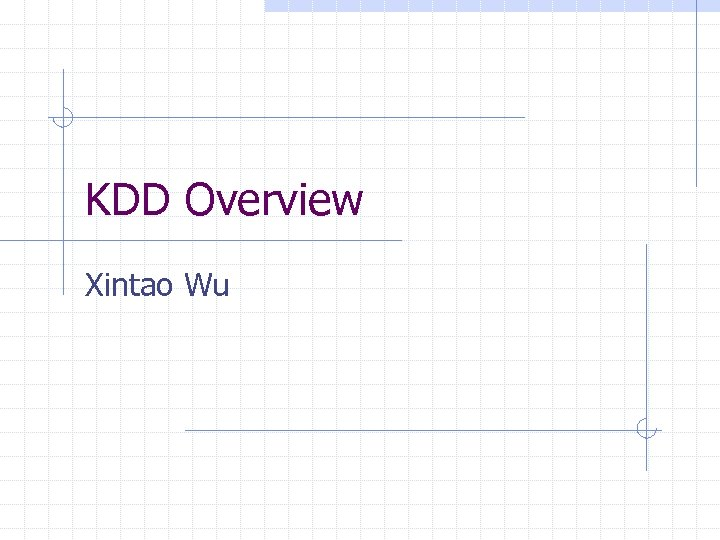KDD Overview Xintao Wu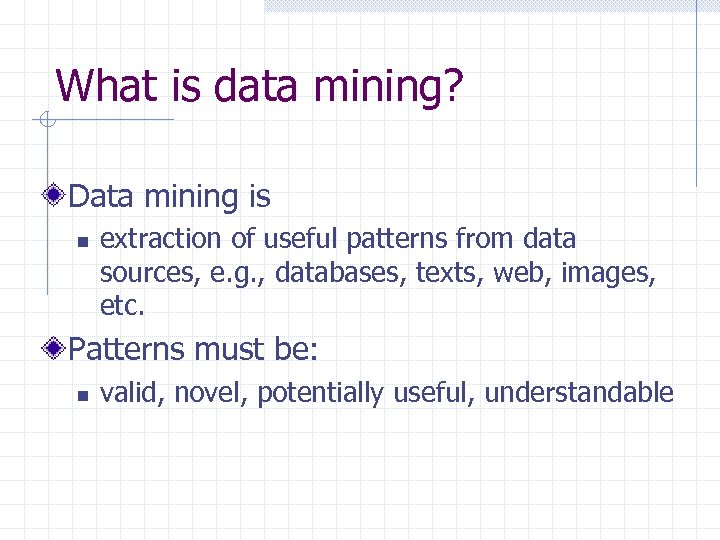What is data mining? Data mining is n extraction of useful patterns from data sources, e. g. , databases, texts, web, images, etc. Patterns must be: n valid, novel, potentially useful, understandable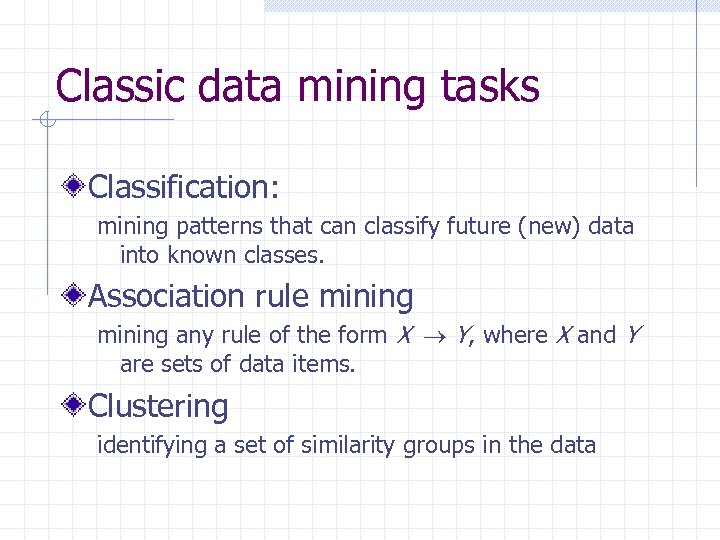Classic data mining tasks Classification: mining patterns that can classify future (new) data into known classes. Association rule mining any rule of the form X Y, where X and Y are sets of data items. Clustering identifying a set of similarity groups in the data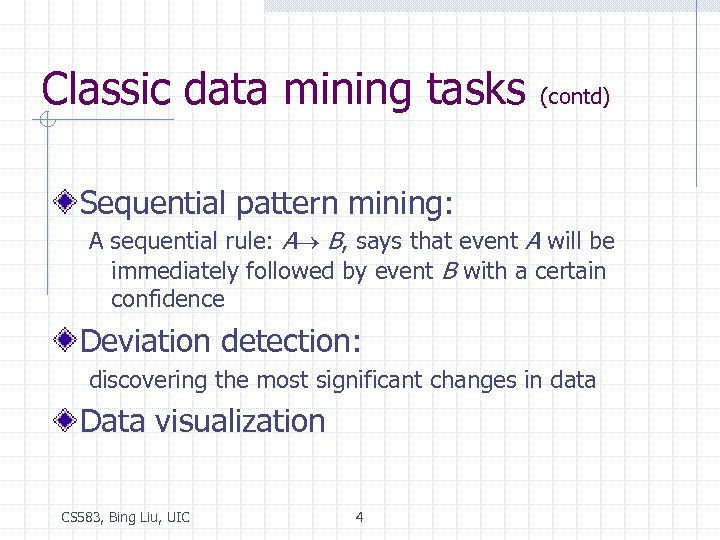Classic data mining tasks (contd) Sequential pattern mining: A sequential rule: A B, says that event A will be immediately followed by event B with a certain confidence Deviation detection: discovering the most significant changes in data Data visualization CS 583, Bing Liu, UIC 4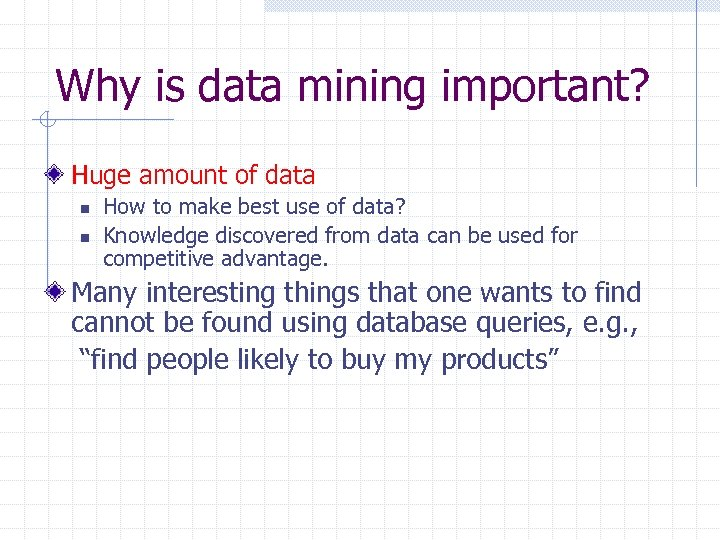Why is data mining important? Huge amount of data n n How to make best use of data? Knowledge discovered from data can be used for competitive advantage. Many interesting things that one wants to find cannot be found using database queries, e. g. , “find people likely to buy my products”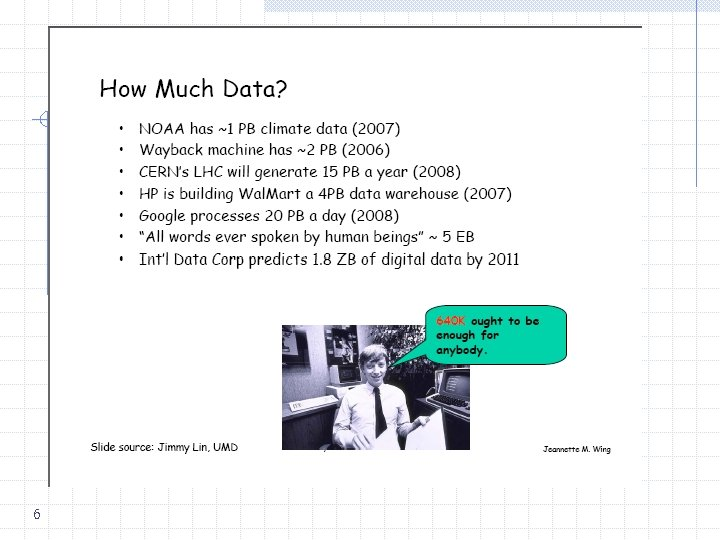6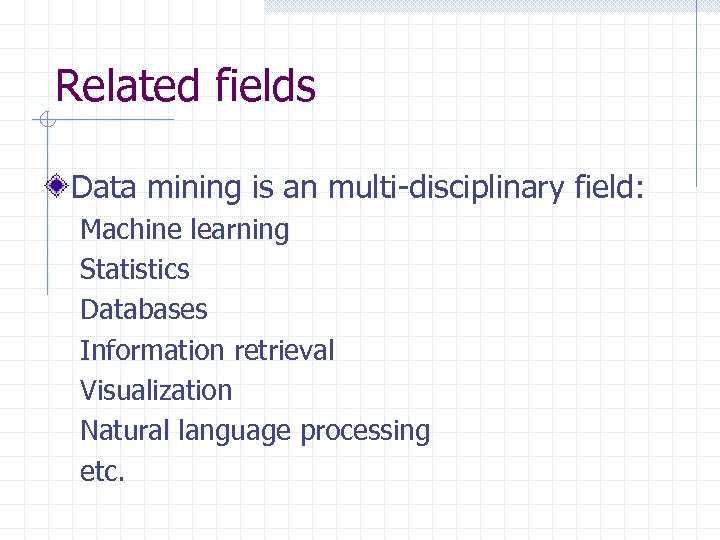Related fields Data mining is an multi-disciplinary field: Machine learning Statistics Databases Information retrieval Visualization Natural language processing etc.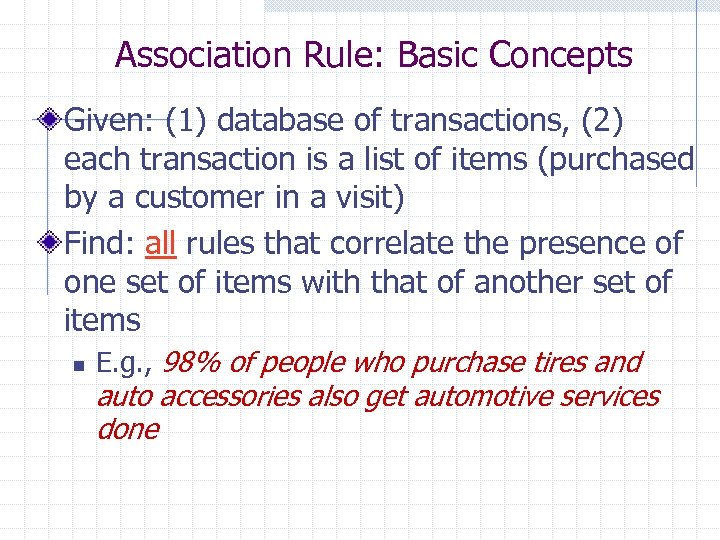Association Rule: Basic Concepts Given: (1) database of transactions, (2) each transaction is a list of items (purchased by a customer in a visit) Find: all rules that correlate the presence of one set of items with that of another set of items n E. g. , 98% of people who purchase tires and auto accessories also get automotive services done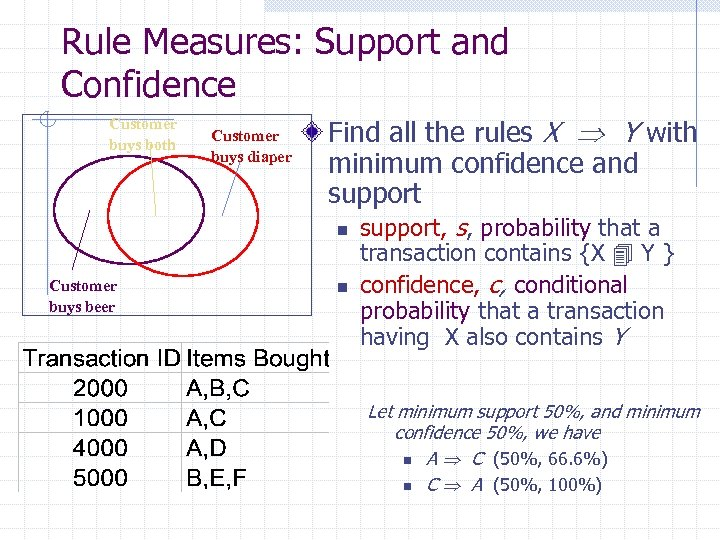Rule Measures: Support and Confidence Customer buys both Customer buys diaper Find all the rules X Y with minimum confidence and support n Customer buys beer n support, s, probability that a transaction contains {X Y } confidence, c, conditional probability that a transaction having X also contains Y Let minimum support 50%, and minimum confidence 50%, we have n A C (50%, 66. 6%) n C A (50%, 100%)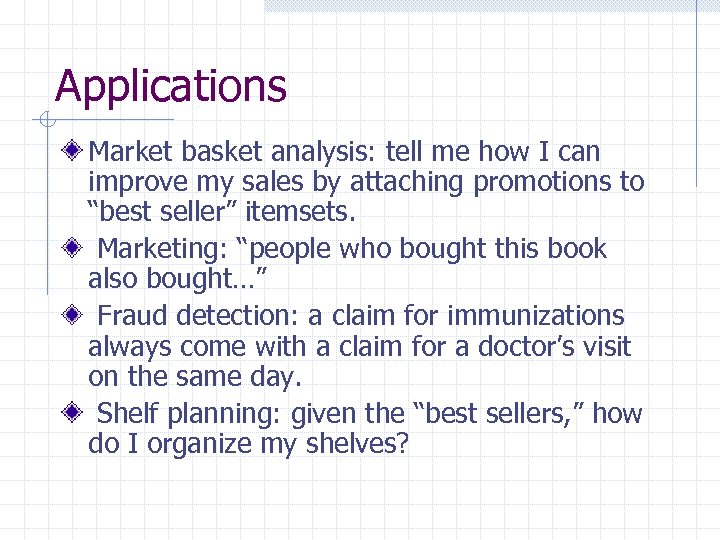Applications Market basket analysis: tell me how I can improve my sales by attaching promotions to “best seller” itemsets. Marketing: “people who bought this book also bought…” Fraud detection: a claim for immunizations always come with a claim for a doctor’s visit on the same day. Shelf planning: given the “best sellers, ” how do I organize my shelves?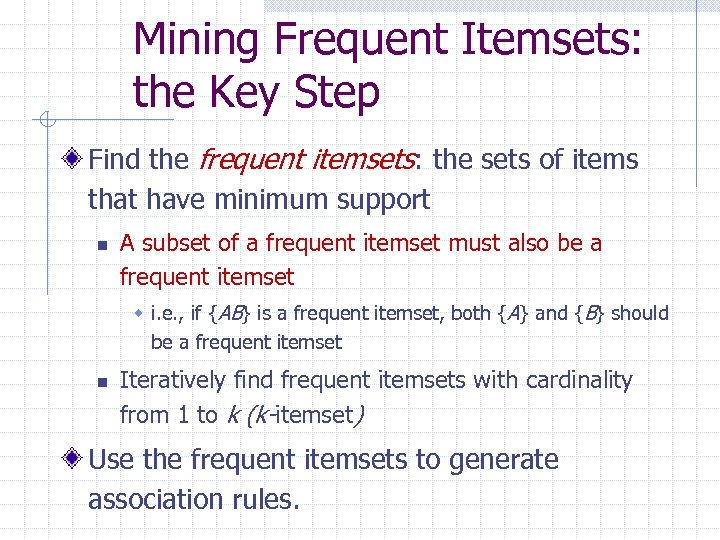Mining Frequent Itemsets: the Key Step Find the frequent itemsets: the sets of items that have minimum support n A subset of a frequent itemset must also be a frequent itemset w i. e. , if {AB} is a frequent itemset, both {A} and {B} should be a frequent itemset n Iteratively find frequent itemsets with cardinality from 1 to k (k-itemset) Use the frequent itemsets to generate association rules.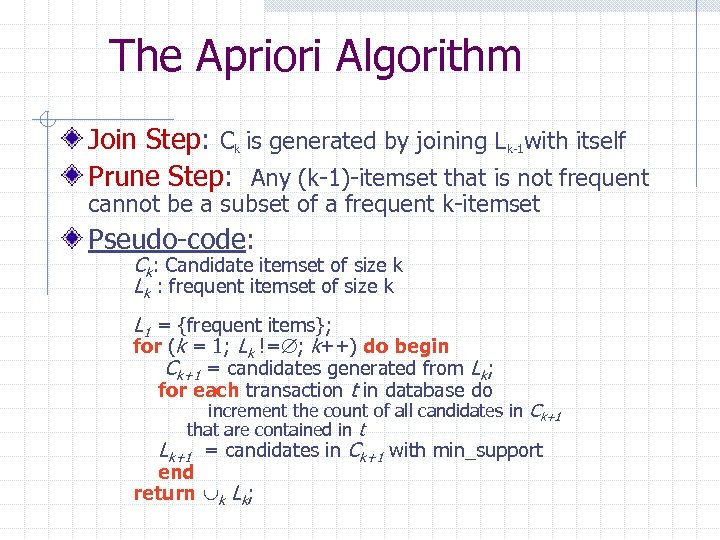The Apriori Algorithm Join Step: Ck is generated by joining Lk-1 with itself Prune Step: Any (k-1)-itemset that is not frequent cannot be a subset of a frequent k-itemset Pseudo-code: Ck: Candidate itemset of size k Lk : frequent itemset of size k L 1 = {frequent items}; for (k = 1; Lk != ; k++) do begin Ck+1 = candidates generated from Lk; for each transaction t in database do increment the count of all candidates in Ck+1 that are contained in t Lk+1 = candidates in Ck+1 with min_support end return k Lk;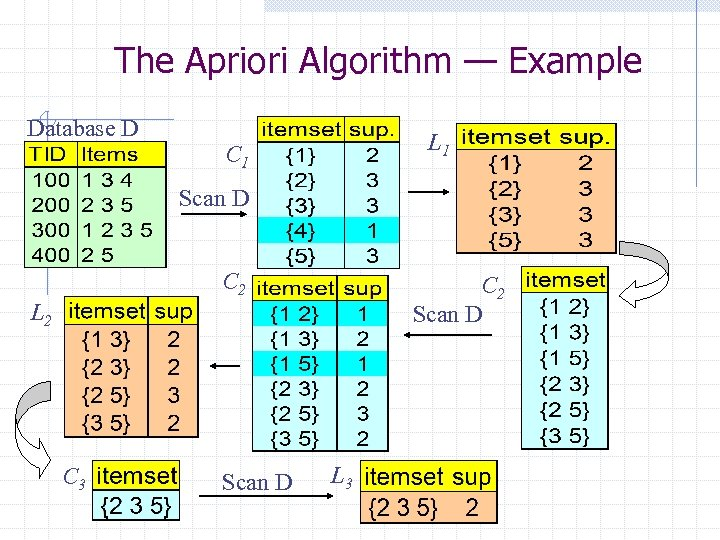The Apriori Algorithm — Example Database D L 1 C 1 Scan D C 2 Scan D L 2 C 3 Scan D L 3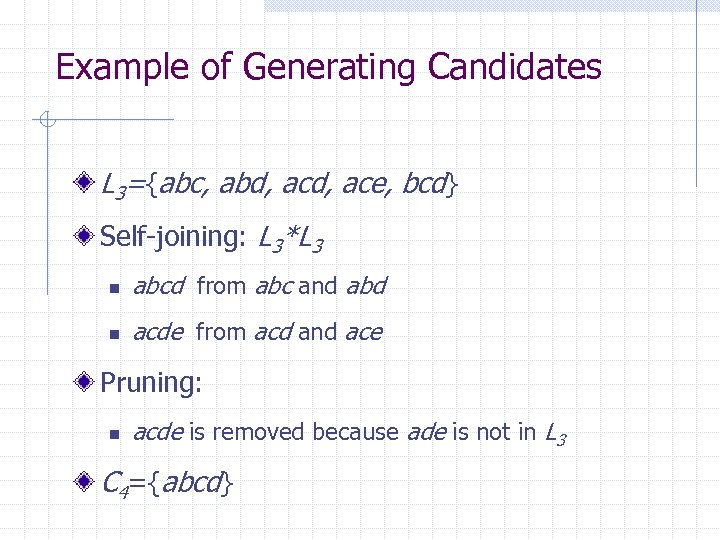Example of Generating Candidates L 3={abc, abd, ace, bcd} Self-joining: L 3*L 3 n abcd from abc and abd n acde from acd and ace Pruning: n acde is removed because ade is not in L 3 C 4={abcd}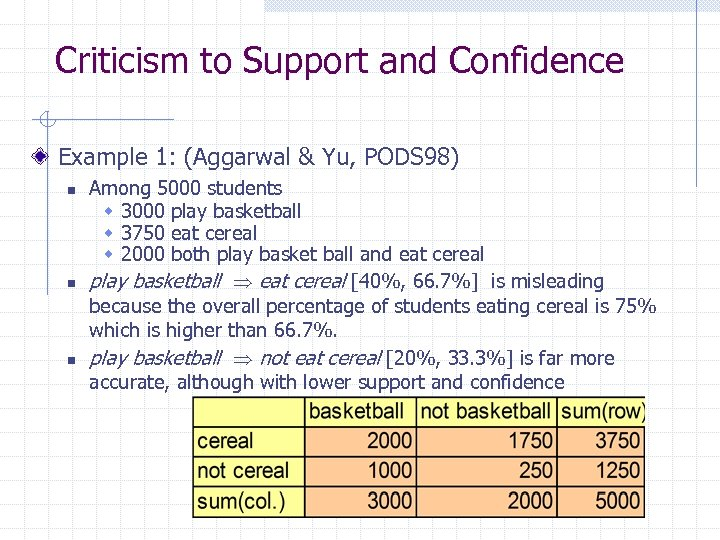Criticism to Support and Confidence Example 1: (Aggarwal & Yu, PODS 98) n n n Among 5000 students w 3000 play basketball w 3750 eat cereal w 2000 both play basket ball and eat cereal play basketball eat cereal [40%, 66. 7%] is misleading because the overall percentage of students eating cereal is 75% which is higher than 66. 7%. play basketball not eat cereal [20%, 33. 3%] is far more accurate, although with lower support and confidence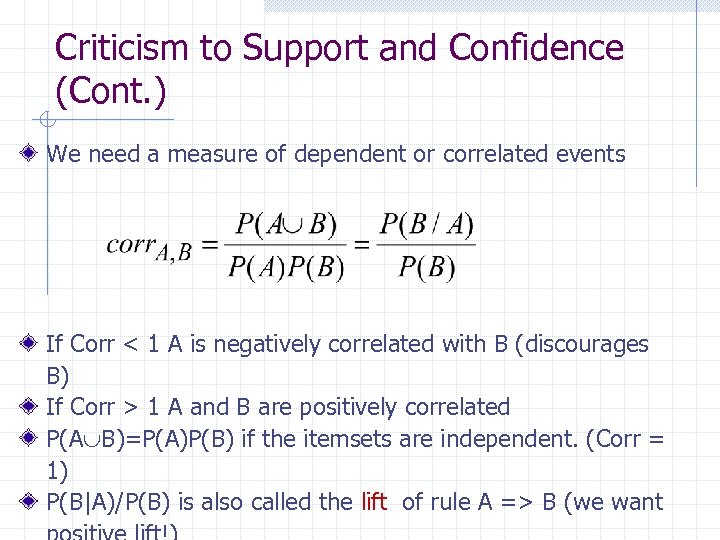Criticism to Support and Confidence (Cont. ) We need a measure of dependent or correlated events If Corr < 1 A is negatively correlated with B (discourages B) If Corr > 1 A and B are positively correlated P(A B)=P(A)P(B) if the itemsets are independent. (Corr = 1) P(B|A)/P(B) is also called the lift of rule A => B (we want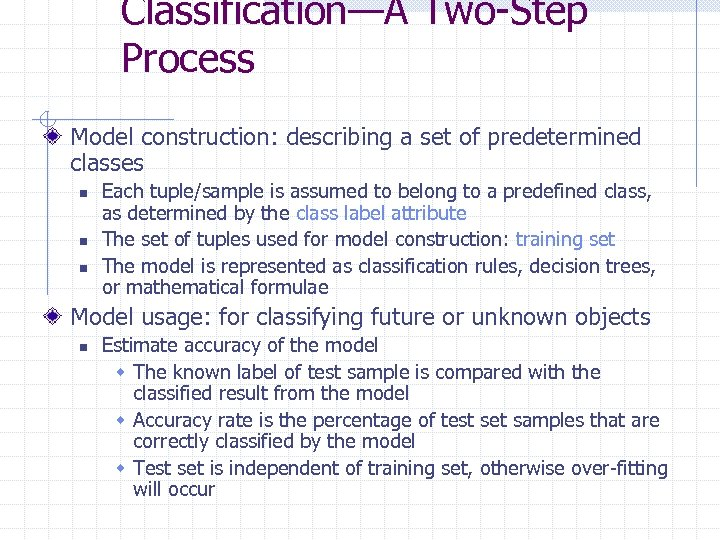Classification—A Two-Step Process Model construction: describing a set of predetermined classes n n n Each tuple/sample is assumed to belong to a predefined class, as determined by the class label attribute The set of tuples used for model construction: training set The model is represented as classification rules, decision trees, or mathematical formulae Model usage: for classifying future or unknown objects n Estimate accuracy of the model w The known label of test sample is compared with the classified result from the model w Accuracy rate is the percentage of test set samples that are correctly classified by the model w Test set is independent of training set, otherwise over-fitting will occur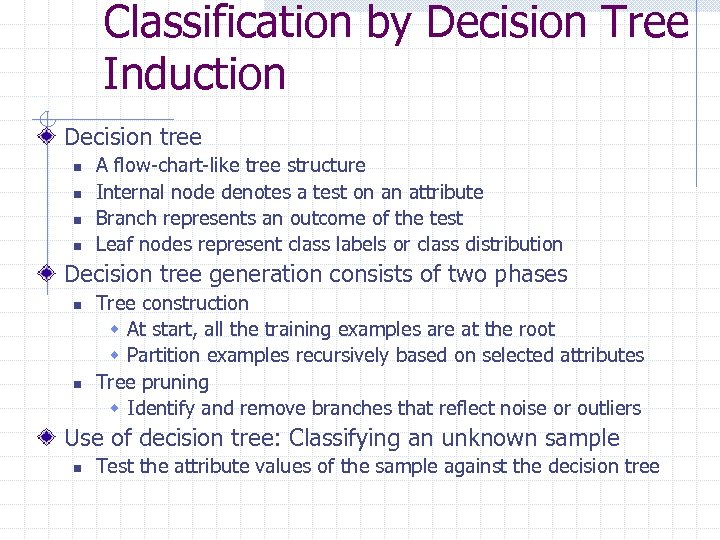Classification by Decision Tree Induction Decision tree n n A flow-chart-like tree structure Internal node denotes a test on an attribute Branch represents an outcome of the test Leaf nodes represent class labels or class distribution Decision tree generation consists of two phases n n Tree construction w At start, all the training examples are at the root w Partition examples recursively based on selected attributes Tree pruning w Identify and remove branches that reflect noise or outliers Use of decision tree: Classifying an unknown sample n Test the attribute values of the sample against the decision tree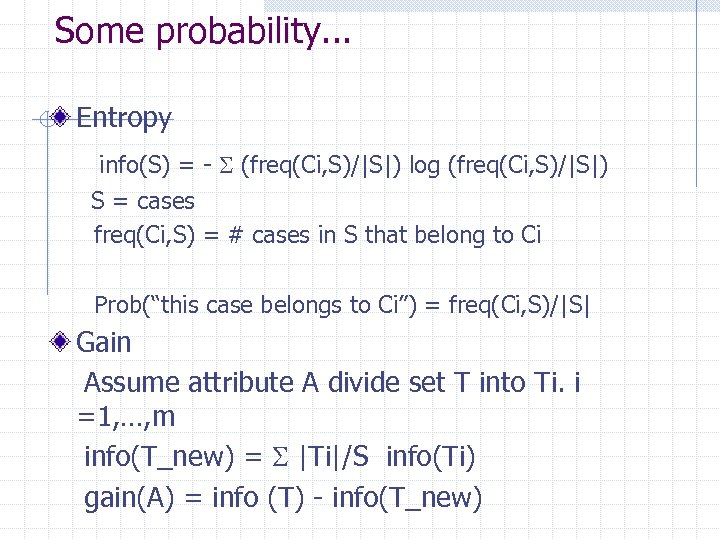Some probability. . . Entropy info(S) = - (freq(Ci, S)/|S|) log (freq(Ci, S)/|S|) S = cases freq(Ci, S) = # cases in S that belong to Ci Prob(“this case belongs to Ci”) = freq(Ci, S)/|S| Gain Assume attribute A divide set T into Ti. i =1, …, m info(T_new) = |Ti|/S info(Ti) gain(A) = info (T) - info(T_new)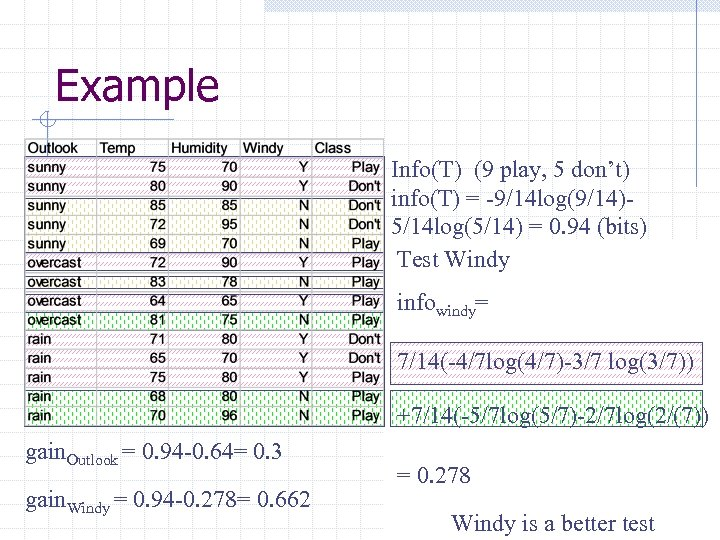Example Info(T) (9 play, 5 don’t) info(T) = -9/14 log(9/14)5/14 log(5/14) = 0. 94 (bits) Test outlook Test: Windy infowindy= info. Outlook = gain. Outlook = 0. 94 -0. 64= 0. 3 gain. Windy = 0. 94 -0. 278= 0. 662 5/14 (-2/5 log(2/5)-3/5 log(3/5))+ 7/14(-4/7 log(4/7)-3/7 log(3/7)) 4/14 (-4/4 log(4/4)) + +7/14(-5/7 log(5/7)-2/7 log(2/(7)) 5/14 (-3/5 log(3/5) - 2/5 log(2/5)) = 0. 278 = 0. 64 (bits) Windy is a better test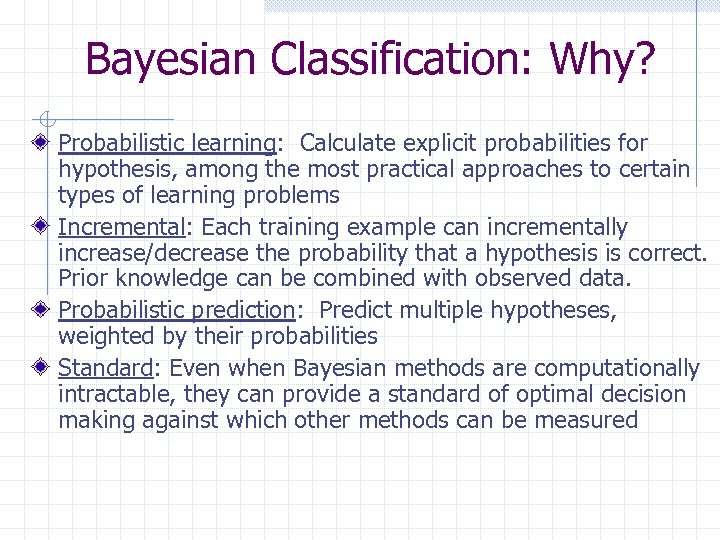Bayesian Classification: Why? Probabilistic learning: Calculate explicit probabilities for hypothesis, among the most practical approaches to certain types of learning problems Incremental: Each training example can incrementally increase/decrease the probability that a hypothesis is correct. Prior knowledge can be combined with observed data. Probabilistic prediction: Predict multiple hypotheses, weighted by their probabilities Standard: Even when Bayesian methods are computationally intractable, they can provide a standard of optimal decision making against which other methods can be measured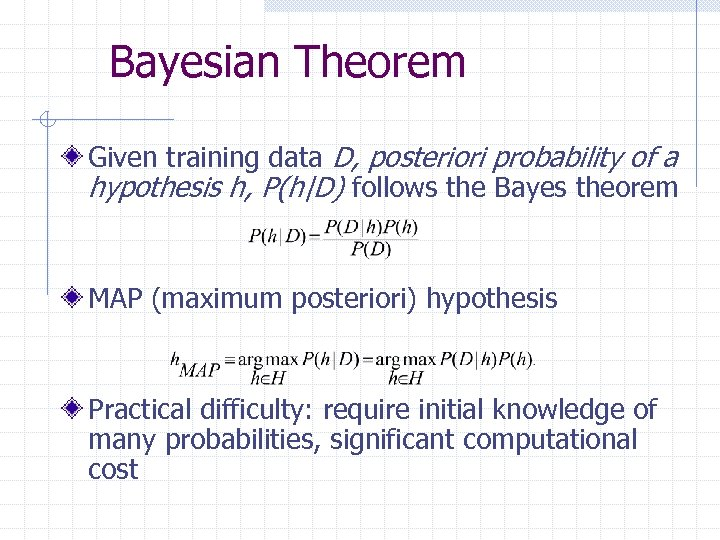Bayesian Theorem Given training data D, posteriori probability of a hypothesis h, P(h|D) follows the Bayes theorem MAP (maximum posteriori) hypothesis Practical difficulty: require initial knowledge of many probabilities, significant computational cost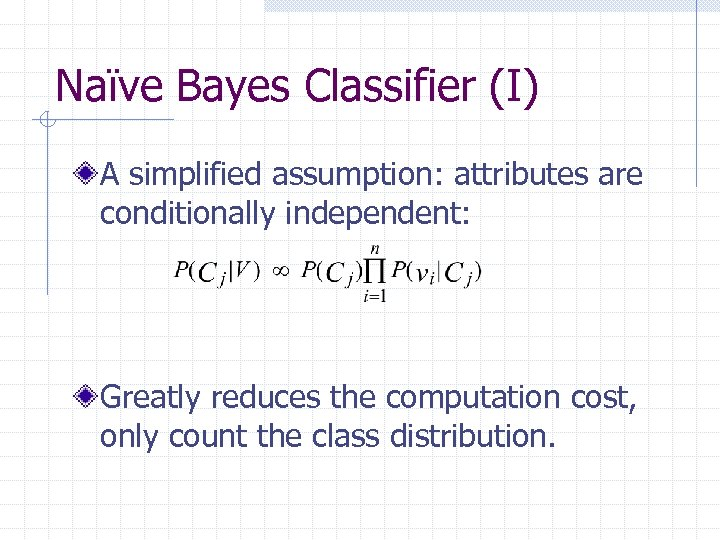Naïve Bayes Classifier (I) A simplified assumption: attributes are conditionally independent: Greatly reduces the computation cost, only count the class distribution.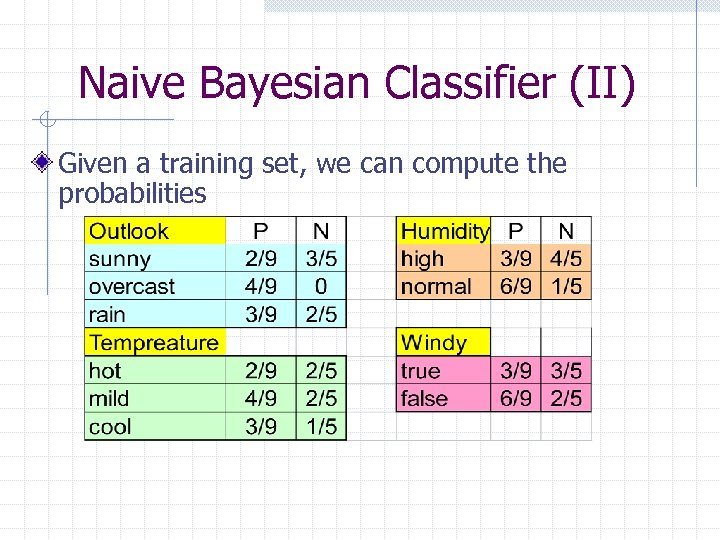Naive Bayesian Classifier (II) Given a training set, we can compute the probabilities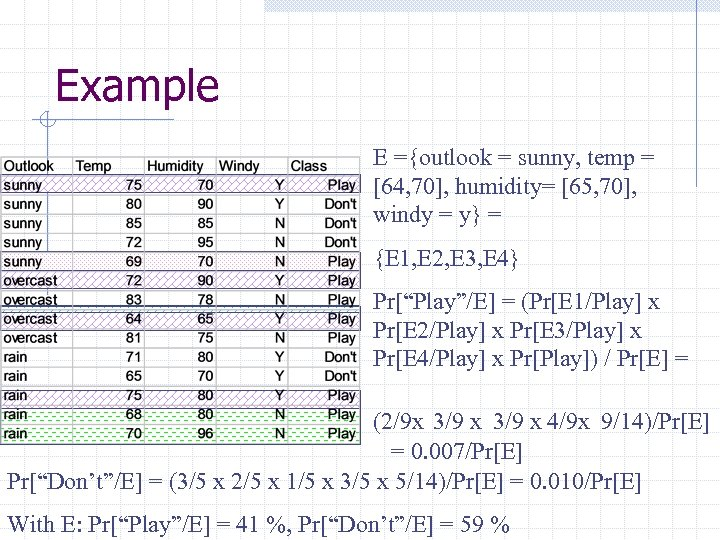Example E ={outlook = sunny, temp = [64, 70], humidity= [65, 70], windy = y} = {E 1, E 2, E 3, E 4} Pr[“Play”/E] = (Pr[E 1/Play] x Pr[E 2/Play] x Pr[E 3/Play] x Pr[E 4/Play] x Pr[Play]) / Pr[E] = (2/9 x 3/9 x 4/9 x 9/14)/Pr[E] = 0. 007/Pr[E] Pr[“Don’t”/E] = (3/5 x 2/5 x 1/5 x 3/5 x 5/14)/Pr[E] = 0. 010/Pr[E] With E: Pr[“Play”/E] = 41 %, Pr[“Don’t”/E] = 59 %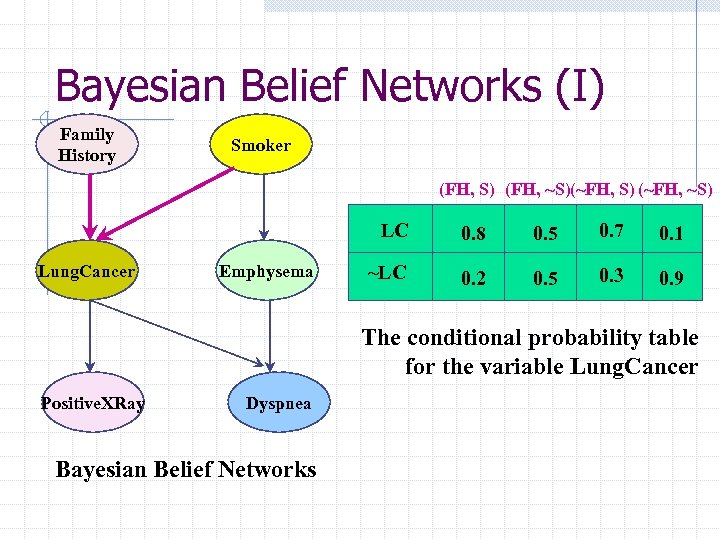Bayesian Belief Networks (I) Family History Smoker (FH, S) (FH, ~S)(~FH, S) (~FH, ~S) LC Lung. Cancer Emphysema 0. 8 0. 5 0. 7 0. 1 ~LC 0. 2 0. 5 0. 3 0. 9 The conditional probability table for the variable Lung. Cancer Positive. XRay Dyspnea Bayesian Belief Networks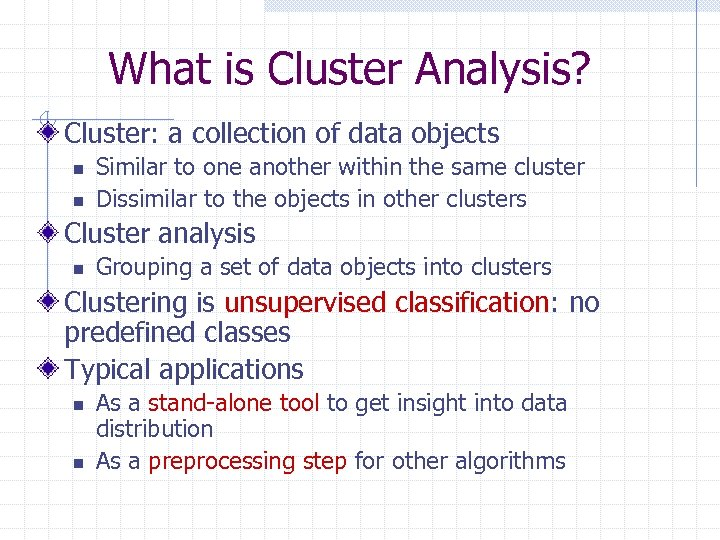What is Cluster Analysis? Cluster: a collection of data objects n n Similar to one another within the same cluster Dissimilar to the objects in other clusters Cluster analysis n Grouping a set of data objects into clusters Clustering is unsupervised classification: no predefined classes Typical applications n n As a stand-alone tool to get insight into data distribution As a preprocessing step for other algorithms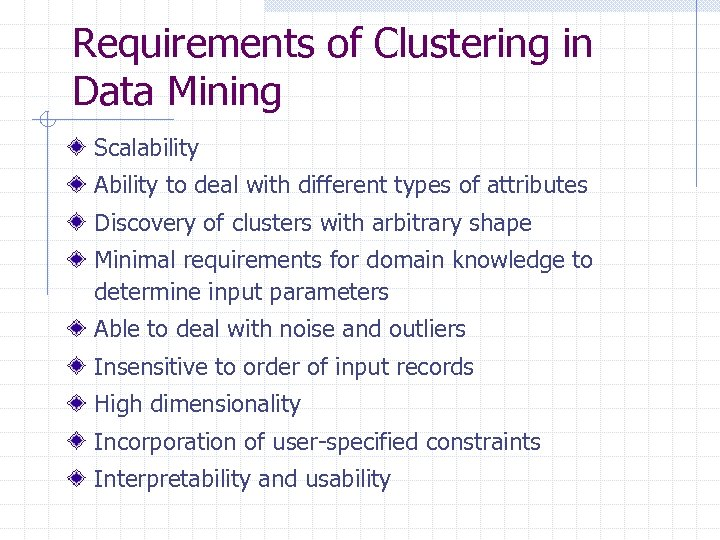Requirements of Clustering in Data Mining Scalability Ability to deal with different types of attributes Discovery of clusters with arbitrary shape Minimal requirements for domain knowledge to determine input parameters Able to deal with noise and outliers Insensitive to order of input records High dimensionality Incorporation of user-specified constraints Interpretability and usability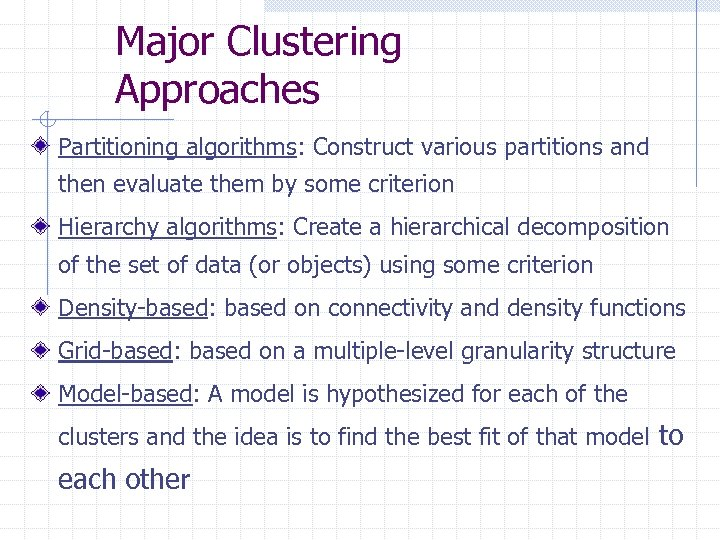Major Clustering Approaches Partitioning algorithms: Construct various partitions and then evaluate them by some criterion Hierarchy algorithms: Create a hierarchical decomposition of the set of data (or objects) using some criterion Density-based: based on connectivity and density functions Grid-based: based on a multiple-level granularity structure Model-based: A model is hypothesized for each of the clusters and the idea is to find the best fit of that model to each other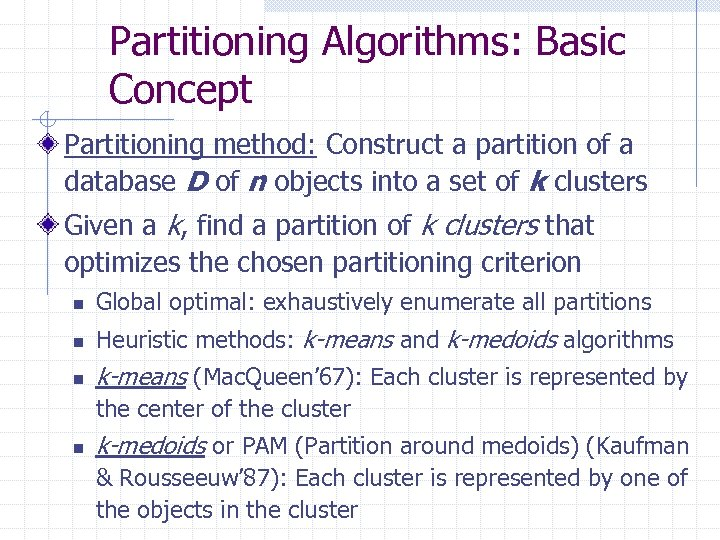Partitioning Algorithms: Basic Concept Partitioning method: Construct a partition of a database D of n objects into a set of k clusters Given a k, find a partition of k clusters that optimizes the chosen partitioning criterion n Global optimal: exhaustively enumerate all partitions n Heuristic methods: k-means and k-medoids algorithms n k-means (Mac. Queen’ 67): Each cluster is represented by the center of the cluster n k-medoids or PAM (Partition around medoids) (Kaufman & Rousseeuw’ 87): Each cluster is represented by one of the objects in the cluster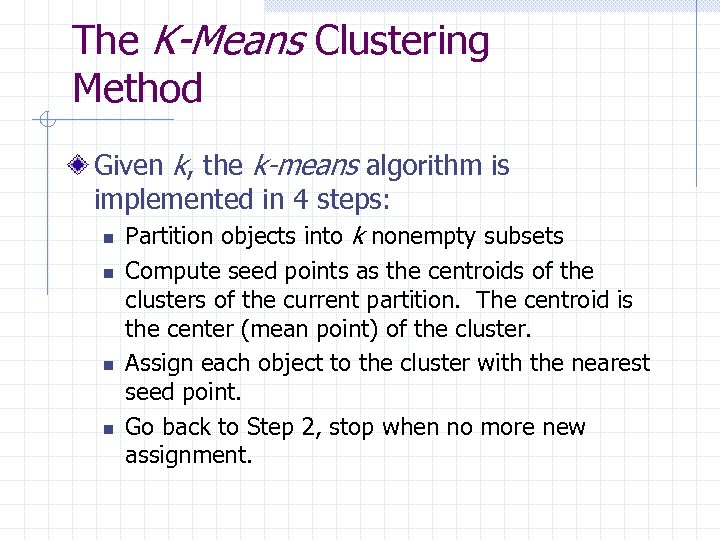The K-Means Clustering Method Given k, the k-means algorithm is implemented in 4 steps: n n Partition objects into k nonempty subsets Compute seed points as the centroids of the clusters of the current partition. The centroid is the center (mean point) of the cluster. Assign each object to the cluster with the nearest seed point. Go back to Step 2, stop when no more new assignment.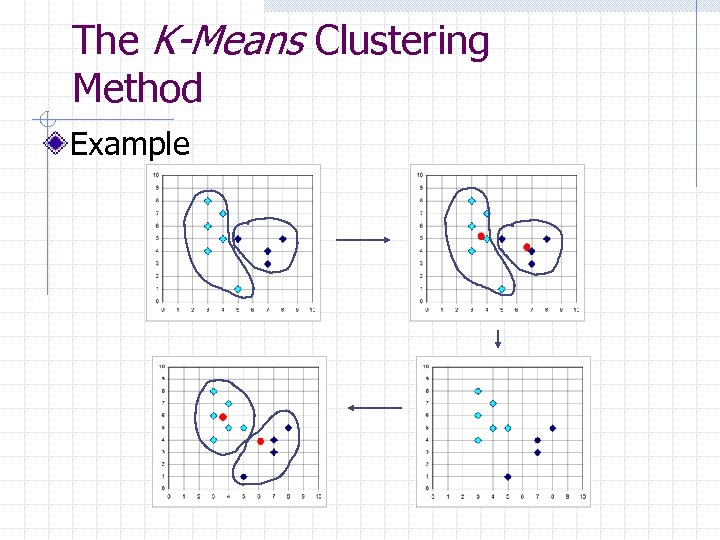The K-Means Clustering Method Example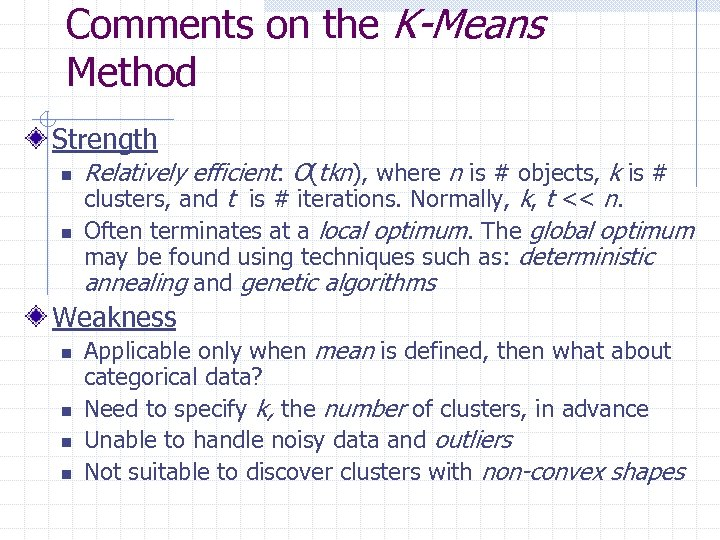Comments on the K-Means Method Strength n n Relatively efficient: O(tkn), where n is # objects, k is # clusters, and t is # iterations. Normally, k, t << n. Often terminates at a local optimum. The global optimum may be found using techniques such as: deterministic annealing and genetic algorithms Weakness n n Applicable only when mean is defined, then what about categorical data? Need to specify k, the number of clusters, in advance Unable to handle noisy data and outliers Not suitable to discover clusters with non-convex shapes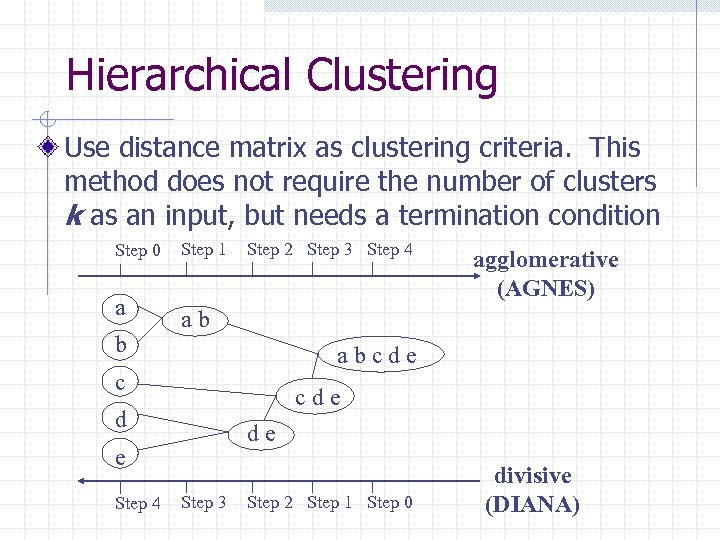Hierarchical Clustering Use distance matrix as clustering criteria. This method does not require the number of clusters k as an input, but needs a termination condition Step 0 a b Step 1 Step 2 Step 3 Step 4 ab abcde c cde d de e Step 4 agglomerative (AGNES) Step 3 Step 2 Step 1 Step 0 divisive (DIANA)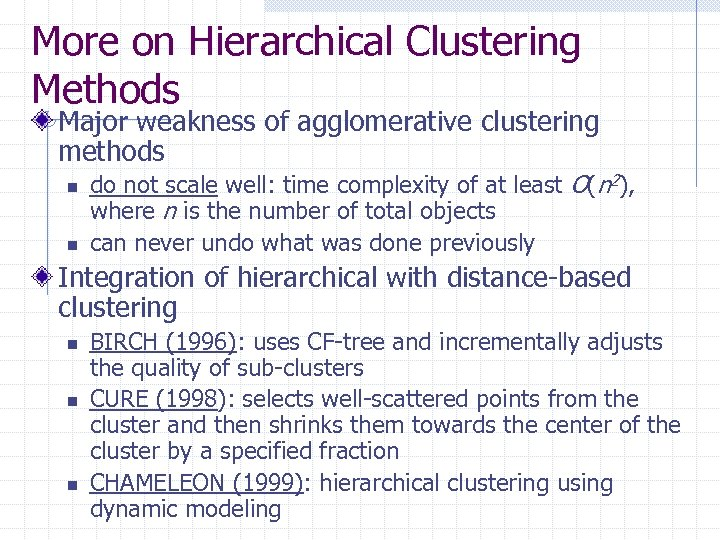More on Hierarchical Clustering Methods Major weakness of agglomerative clustering methods n n do not scale well: time complexity of at least O(n 2), where n is the number of total objects can never undo what was done previously Integration of hierarchical with distance-based clustering n n n BIRCH (1996): uses CF-tree and incrementally adjusts the quality of sub-clusters CURE (1998): selects well-scattered points from the cluster and then shrinks them towards the center of the cluster by a specified fraction CHAMELEON (1999): hierarchical clustering using dynamic modeling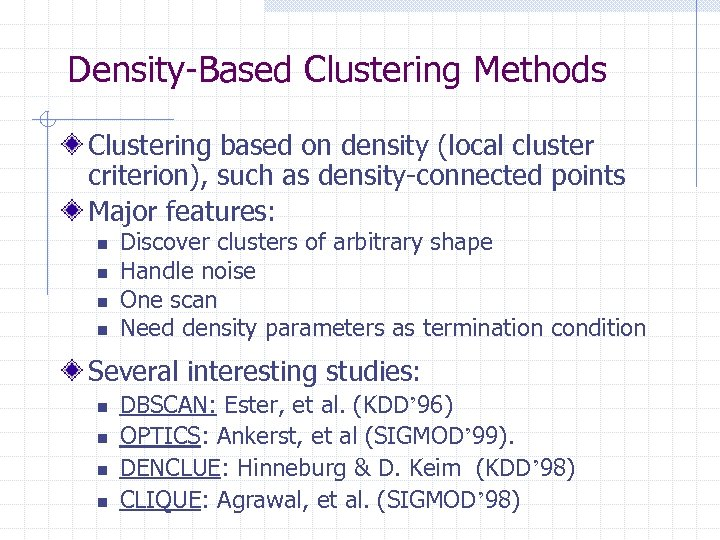Density-Based Clustering Methods Clustering based on density (local cluster criterion), such as density-connected points Major features: n n Discover clusters of arbitrary shape Handle noise One scan Need density parameters as termination condition Several interesting studies: n n DBSCAN: Ester, et al. (KDD’ 96) OPTICS: Ankerst, et al (SIGMOD’ 99). DENCLUE: Hinneburg & D. Keim (KDD’ 98) CLIQUE: Agrawal, et al. (SIGMOD’ 98)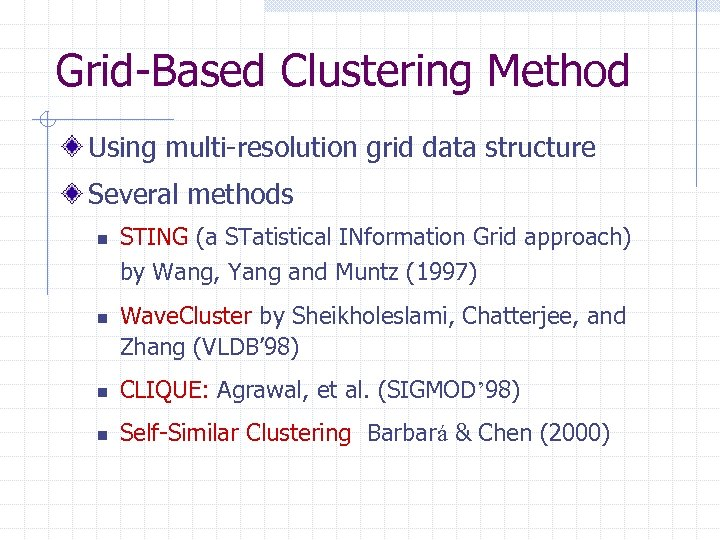Grid-Based Clustering Method Using multi-resolution grid data structure Several methods n n STING (a STatistical INformation Grid approach) by Wang, Yang and Muntz (1997) Wave. Cluster by Sheikholeslami, Chatterjee, and Zhang (VLDB’ 98) n CLIQUE: Agrawal, et al. (SIGMOD’ 98) n Self-Similar Clustering Barbará & Chen (2000)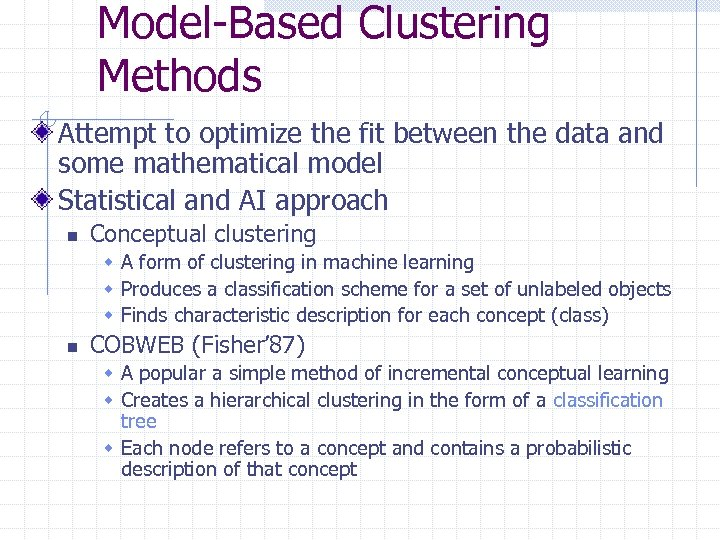Model-Based Clustering Methods Attempt to optimize the fit between the data and some mathematical model Statistical and AI approach n Conceptual clustering w A form of clustering in machine learning w Produces a classification scheme for a set of unlabeled objects w Finds characteristic description for each concept (class) n COBWEB (Fisher’ 87) w A popular a simple method of incremental conceptual learning w Creates a hierarchical clustering in the form of a classification tree w Each node refers to a concept and contains a probabilistic description of that concept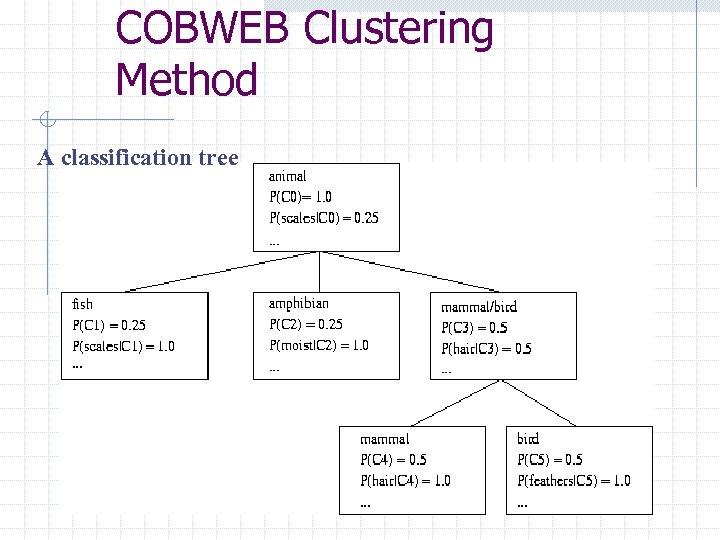COBWEB Clustering Method A classification tree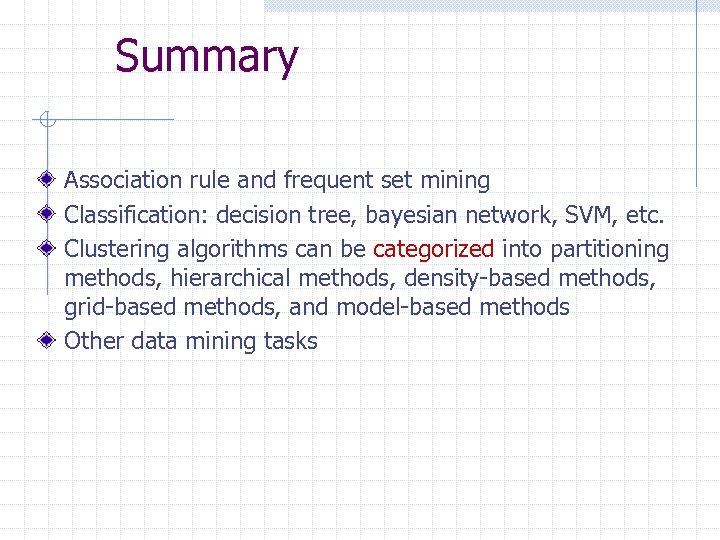Summary Association rule and frequent set mining Classification: decision tree, bayesian network, SVM, etc. Clustering algorithms can be categorized into partitioning methods, hierarchical methods, density-based methods, grid-based methods, and model-based methods Other data mining tasks